﻿ 考虑轴向力作用结构自振频率的简化计算 Simplified Calculation of Natural Frequency of Structure Considering Axial Force

Hans Journal of Civil Engineering
Vol. 08  No. 03 ( 2019 ), Article ID: 30220 , 6 pages
10.12677/HJCE.2019.83072

Simplified Calculation of Natural Frequency of Structure Considering Axial Force

Mengzeng Cao, Bin Li

Civil Engineering School, Inner Mongolia University of Science & Technology, Baotou Inner Mongolia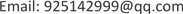Received: Apr. 24th, 2019; accepted: May 9th, 2019; published: May 16th, 2019ABSTRACT

The effect of the axial force has a great influence on the stiffness of the system, because the bending of the bar and the axial force will increase the additional bending moment and increase the geometric stiffness of the structure, the calculation is very complicated after introducing the geometric stiffness.

Keywords:Axial Force, Natural Frequency, Arch Truss, Simplified Calculation1. 引言

2. 简化模型

2.1. 简化模型I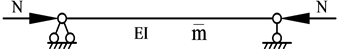Figure 1. Diagram of simply supported beam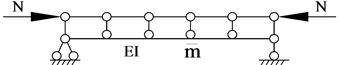Figure 2. Diagram of simply supported beam truss system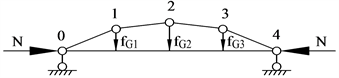Figure 3. Post-deformation diagram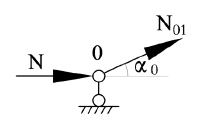Figure 4. Node zero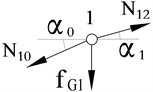Figure 5. Node one

$\sum {F}_{y}=0和\sum {F}_{X}=0$ 得：

${N}_{01}\mathrm{sin}{\alpha }_{0}+{f}_{G1}-{N}_{12}\mathrm{sin}{\alpha }_{1}=0$${N}_{12}\mathrm{cos}{\alpha }_{1}-{N}_{01}\mathrm{cos}{\alpha }_{0}=0$

$-\frac{N}{\mathrm{cos}{\alpha }_{0}}\mathrm{sin}{\alpha }_{0}+{f}_{G1}-\left(-\frac{N}{\mathrm{cos}{\alpha }_{1}}\right)\mathrm{sin}{\alpha }_{1}=0$${f}_{G1}=N\left(\mathrm{tan}{\alpha }_{0}-\mathrm{tan}{\alpha }_{1}\right)$

${f}_{G1}=N\left({\alpha }_{0}-{\alpha }_{1}\right)$ (1)Figure 6. Diagram of calculation under vertical force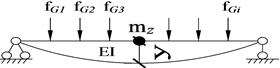Figure 7. Calculation diagram of concentrated mass

$y\left(x\right)=-{m}_{z}\stackrel{¨}{y}\left(x\right){\delta }_{11}+\underset{i=1}{\overset{n}{\sum }}{f}_{Gi}{\delta }_{1i}$ (2)

$\omega =\sqrt{\frac{1}{{m}_{z}}\left(\frac{1}{{\delta }_{11}}+\frac{\underset{i=1}{\overset{n}{\sum }}{f}_{Gi}{\delta }_{1i}}{Y\left(t\right){\delta }_{11}}\right)}$ (3)

2.2. 简化模型II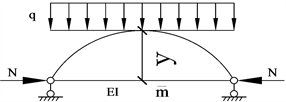Figure 8. Sketch of truss model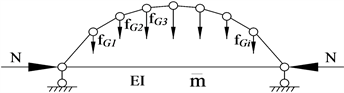Figure 9. Diagram of calculation under uniformly distributed load

$\frac{1}{2}ql\cdot \frac{l}{2}=Ny+q\cdot \frac{l}{2}\cdot \frac{l}{4}$

$q=\frac{8N}{{l}^{2}}y$

$y=\frac{1}{2}\stackrel{¯}{m}l\cdot \stackrel{¨}{y}{\delta }_{11}-\frac{5{l}^{4}}{384}\cdot \frac{8N}{{l}^{2}}y+y=0$

3. 实例计算

$Y\left(x\right)=y\mathrm{sin}\frac{\text{π}}{l}x$ (4)

$1\left\{\begin{array}{l}{\alpha }_{0}=\frac{y\mathrm{sin}\frac{\text{π}}{l}\cdot \frac{l}{4}}{\frac{l}{4}}=\frac{0.707y}{\frac{l}{4}}=\frac{2.828y}{l}\\ {\alpha }_{1}=\frac{\left(1-0.707\right)y}{\frac{l}{4}}=\frac{1.172y}{l}\end{array}$ $2\left\{\begin{array}{l}{\alpha }_{0}=\frac{1.172y}{l}\\ {\alpha }_{1}=-\frac{1.172y}{l}\end{array}$ $3\left\{\begin{array}{l}{\alpha }_{0}=-\frac{1.172y}{l}\\ {\alpha }_{1}=-\frac{2.828y}{l}\end{array}$

${f}_{G1}=N\left(2.828-1.172\right)\frac{y}{l}=1.656\frac{Ny}{l}$

${f}_{G2}=N\left(1.172+1.172\right)\frac{y}{l}=2.344\frac{Ny}{l}$

${f}_{G3}=N\left(-1.172+2.828\right)\frac{y}{l}=1.656\frac{Ny}{l}$

$y=-m\stackrel{¨}{y}{\delta }_{11}+2{f}_{G1}{\delta }_{12}+{f}_{G2}{\delta }_{11}$

${\delta }_{11}=\frac{{l}^{3}}{48EI}，{\delta }_{12}=\frac{11{l}^{3}}{768EI}$

$m\stackrel{¨}{y}+\left(\frac{48EI}{{l}^{3}}-4.62\frac{N}{l}\right)y=0$

$\omega =\frac{{\text{π}}^{2}}{{l}^{2}}\sqrt{\left(1-\frac{0.95N{l}^{2}}{{\pi }^{2}EI}\right)\cdot \frac{EI}{\stackrel{¯}{m}}}$ (5)

$y=\frac{1}{2}\stackrel{¯}{m}l\cdot \stackrel{¨}{y}{\delta }_{11}-\frac{5{l}^{4}}{384}\cdot \frac{8N}{{l}^{2}}y+y=0$

$\omega =\frac{{\text{π}}^{2}}{{l}^{2}}\sqrt{\left(1-\frac{1.027N{l}^{2}}{{\text{π}}^{2}EI}\right)\cdot \frac{EI}{\stackrel{¯}{m}}}$ (6)

$\omega =\frac{{\text{π}}^{2}}{{l}^{2}}\sqrt{\left(1-\frac{N{l}^{2}}{{\text{π}}^{2}EI}\right)\cdot \frac{EI}{\stackrel{¯}{m}}}$ (7)

(7)比值为β1，式(6)与式(7)比值为β2

${\beta }_{1}=\frac{\frac{{\text{π}}^{2}}{{l}^{2}}\sqrt{\left(1-\frac{0.95N{l}^{2}}{{\text{π}}^{2}EI}\right)\cdot \frac{EI}{\stackrel{¯}{m}}}}{\frac{{\text{π}}^{2}}{{l}^{2}}\sqrt{\left(1-\frac{N{l}^{2}}{{\text{π}}^{2}EI}\right)\cdot \frac{EI}{\stackrel{¯}{m}}}}=\frac{1-0.95\theta }{1-\theta }$

${\beta }_{2}=\frac{\frac{{\text{π}}^{2}}{{l}^{2}}\sqrt{\left(1-\frac{1.027N{l}^{2}}{{\text{π}}^{2}EI}\right)\cdot \frac{EI}{\stackrel{¯}{m}}}}{\frac{{\text{π}}^{2}}{{l}^{2}}\sqrt{\left(1-\frac{N{l}^{2}}{{\text{π}}^{2}EI}\right)\cdot \frac{EI}{\stackrel{¯}{m}}}}=\frac{1-1.027\theta }{1-\theta }$

4. 结论

1) 利用简化方法求出的自振频率同分离变量法求出的自振频率进行对比，由于θ值很小，可发现自振频率相差不大，但是计算量却大大减少，提高工作效率。

2) 有些问题可以适当进行简化，不要弄得过于繁琐。例如实际问题中初步计算无铰拱的水平推力大小，就可以转化成三铰拱下求水平推力，经过计算，水平推力大小相差不大。

Simplified Calculation of Natural Frequency of Structure Considering Axial Force[J]. 土木工程, 2019, 08(03): 611-616. https://doi.org/10.12677/HJCE.2019.83072

1. 1. 龙驭球, 包世华, 等. 结构力学I: 基本教程[M]. 第3版. 北京: 高等教育出版社, 2012.

2. 2. 龙驭球, 包世华, 等. 结构力学II: 基本教程[M]. 第3版. 北京: 高等教育出版社, 2012.

3. 3. 包世华. 结构动力学[M]. 武汉: 武汉理工大学出版社, 2017.

4. 4. 刘晶波, 杜修力. 结构动力学[M]. 北京: 机械工业出版社, 2005.